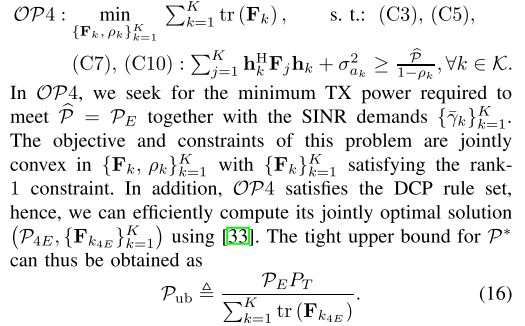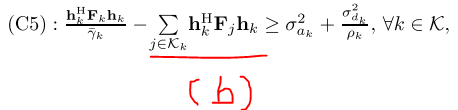# Does anyone have any similar question type for this ? Using DCP ruleset to derive the formula nu using cvx in matlabThe author said that OP4 satisfy the DCP ruleset,so i can obtain the P* ,P_ub, formula like this,so does it means that i just write the lagrange function of OP4 , then i can get the Pub formula ? but don’t i just get a or some value of P_ub instead of formula of P_ub by using the cvx in matlab ?
Do i misunderstand something ? or does anyone have the similar question type and answer like this ?

I won’t address the rank one constraint because it is not shown. I will only address the objective function and the (multiple instances) of the single constraint shown.

After you have read the CVX User’s Guide http://cvxr.com/cvx/doc/, you should see that the problem can be entered essentially as shown in the image you posted, except for the right-hand side of the constraint. You can use
`rho_hat * inv_pos(1- rho(k))`
for that, presuming that you are willing to have the implicit constraint `rho(k) < 1 .`

I have write the code below,but i am a rookie in cvx,so i am not sure that my code is right or wrong.
code

K=4; % channel amount
N=4; % antenna amount in TX
r_k=10; %SINR constraint

nois_var_ak=1;%the variance of the noise ak white noise
nois_var_dk=1;%the variance of the noise dk,%Zero-Mean Circularly Symmetric Complex Gaussian (ZMCSCG) random variables
P_hat=0.00001; %harvested power

h_k=sqrt(1/2)(randn(1,K)+1irandn(1,K));;%AWGN channel

% to do the formula (b)
S=sum(h_k);
H=S-h_k;

%cvx begin

cvx_begin
variable fk(K)
variable fj(j)
Fk=fk * fk’;
Fj=fj * fj’;
variable rho_k(K)
minimize( symsum(abs(Fk)^2,k,1,K) )
subject to
0<=rho(k)<=1;%c3
( h_k’.Fk.h_kinv_pos(r_k) )-sum(mu_k . fk .* H )>= (nois_var_ak)^2+((nois_var_dk)^2*inv_pos(rho(k)) );%c5
Fk >= 0;%c7
symsum(h_k’.*Fj.*h_k,j,1,K)+(nois_var_ak)^2 >= P_hat * inv_pos(1- rho(k));%c10
cvx_endWhy are you using `symsum` (from symbolic toolbox)? Does CVX even accept your program? I can’t test it because I don;t have the symbolic toolbox, but it looks rather dubious to me.

I don’t know whether symsum “works” with CVX, but you should not be using symsum with CVX. If CVX accepts symsum without producing an error message, I have no idea what it is actually doing. You will not find anywhere in the CVX documentation where it says that symsum can be applied to CVX variables or expressions.

Please read the CVX Uers’ Guide http://cvxr.com/cvx/doc/ . As you will see there, sum can be applied to CVX variables and expressions - please use it.

yes!you are right, terrible mistake.
Now the window just told me this,and i am stuck here
Disciplined convex programming error:
Only scalar quadratic forms can be specified in CVX

Error in upper_bound_winter (line 17)
Fk=fk*fk’;

I have already seen your reply about this on the other question,but as i know ,f1 are a value,but fk ,k=1~K, should become a matrix,but you said that we should use a scalar instead of matrix.What should i do

You are not supposed to include `Fk == fk*fk'` as part of the CVX program. That is a non-convex constraint. You are solving some convex relaxation of that. So somewhere there should be a semidefinite constraint on `Fk` rather than the non-convex rank one constraint `Fk == fk*fk'` .

You might want to take a look at the question and my answer at https://scicomp.stackexchange.com/questions/27206/imposing-special-structure-on-positive-semi-definite-matrix/27207#27207 .

but if i add the semidefinite constraint,that is ,

variable fk(K)

become

variable fk(K) semidefinite

The semidefinite needs a square matrix,but in fact ,the paper had said that fk(K) is a N*1 matrix

Only Fk, the square matrix, should appear in your program. Not the vector fk, except if that appears as part of a matrix in a Schur complement formulation, or some such.

1 Like